# Math problem (year 8 level, should be easy)

#### gifi4

##### How am I a 'New Member'?
OP
Member
Well on my text book, the exercise is 7.4 (not relevant but w/e) and the question is "Form an equivalent equation to each of the following by performing the operation given in brackets."

Now the first question.

"x-4=1 (+7)"

Note: the "x" is a pronumeral.

I'm expected to hand this in tomorrow and I have no idea what to do so if someone can help me by explaining how to do it, I will be incredibly grateful.

#### nick22

##### Member
Newcomer
Just add 7 at both sides of the 'is equal to' sign...

#### Scott-105

##### Bow to me. Please?
Member
As the poster above said, add 7 to each side.

The new equation would be: x + 3 = 8

#### Attila13

##### Praise the Creep!
Member
x=5#### Thoob

Member
Yeah, I think he would have preferred the answer over a month ago, when he asked. Nice bump.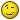#### Attila13

##### Praise the Creep!
Member
Thoob said:
Yeah, I think he would have preferred the answer over a month ago, when he asked. Nice bump.Sorry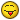I wasn't registered back then!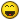#### Thoob

Member
Attila13 said:
Thoob said:
Yeah, I think he would have preferred the answer over a month ago, when he asked. Nice bump.SorryI wasn't registered back then!In fact, from a glance at your profile, you registered a week before then. FAIL.

#### basher11

##### Well-Known Member
Member
>_> you registered on 10/11/10.

the topic was started on 10/18/10#### mameks

##### in memoriam of gravitas
Member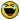Nice bump ;3
At least you can do algebra, even though you can't do dates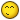#### Attila13

##### Praise the Creep!
Member
Never mind... I was in a rushIt's true that I registered on the 11th October, but then I wasn't very familiar with the forums...
I don't mind you can laugh at me as much as you want, I don't care...We're here to make friends...(I sure am)

P.S.
@ shlong
almost 1000 post dude!#### mameks

##### in memoriam of gravitas
Member
Attila13 said:
Never mind... I was in a rushIt's true that I registered on the 11th October, but then I wasn't very familiar with the forums...
I don't mind you can laugh at me as much as you want, I don't care...We're here to make friends...(I sure am)

P.S.
@ shlong
almost 1000 post dude!I know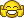Not that I'm going to spam any of it though :3

#### The Pi

##### Lurker
Member
What's the proof of 1+1=2?

#### _Chaz_

##### GBAtemp's Official Mook™
Member
The Pi said:
What's the proof of 1+1=2?
1+1=2, because I told it to.

#### Attila13

##### Praise the Creep!
Member
_Chaz_ said:
The Pi said:
What's the proof of 1+1=2?
1+1=2, because I told it to.
Makes sense!Member

#### hetoan2

##### Well-Known Member
Newcomer
actually i think there is a proof for 1+1=2 which is around 500 pages iirc.

it's easier to prove that .999... = 1

#### tj_cool

##### Site dev
Supervisor
(1 + 1) x 0 = 2 x 0
-> 1 + 1 = 2
Q.E.D.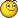#### The Pi

##### Lurker
Member
The actual proof is proving that 2>1 then doing something else which I can't remember.
I have it in my notes somewhere it's 1 page not 500#### ProtoKun7

##### GBAtemp Time Lord Regenerations: 4
Former Staff
tj_cool said:
(1 + 1) x 0 = 2 x 0
-> 1 + 1 = 2
Q.E.D.Now to prove that the universe has no inhabitants.

##### Blade v3+ (I R SHMEXY)
Member
QUOTE said:
The proof starts from the Peano Postulates, which define the natural
numbers N. N is the smallest set satisfying these postulates:

P1. 1 is in N.
P2. If x is in N, then its "successor" x' is in N.
P3. There is no x such that x' = 1.
P4. If x isn't 1, then there is a y in N such that y' = x.
P5. If S is a subset of N, 1 is in S, and the implication
(x in S => x' in S) holds, then S = N.

Then you have to define addition recursively:
Def: Let a and b be in N. If b = 1, then define a + b = a'
(using P1 and P2). If b isn't 1, then let c' = b, with c in N
(using P4), and define a + b = (a + c)'.

Then you have to define 2:
Def: 2 = 1'

2 is in N by P1, P2, and the definition of 2.

Theorem: 1 + 1 = 2

Proof: Use the first part of the definition of + with a = b = 1.
Then 1 + 1 = 1' = 2 Q.E.D.

Note: There is an alternate formulation of the Peano Postulates which
replaces 1 with 0 in P1, P3, P4, and P5. Then you have to change the
definition of addition to this:
Def: Let a and b be in N. If b = 0, then define a + b = a.
If b isn't 0, then let c' = b, with c in N, and define
a + b = (a + c)'.

You also have to define 1 = 0', and 2 = 1'. Then the proof of the
Theorem above is a little different:

Proof: Use the second part of the definition of + first:
1 + 1 = (1 + 0)'
Now use the first part of the definition of + on the sum in
parentheses: 1 + 1 = (1)' = 1' = 2 Q.E.D.

tj_cool said:
(1 + 1) x 0 = 2 x 0
-> 1 + 1 = 2
Q.E.D.So that means that:

(685944.543 + 1043255252.435252) X 0 = 2 X 0
-> 685944.543 + 1043255252.435252 = 2

I highly doubt it.

•••Replies
10
Views
2K
•Replies
6
Views
4K
•Replies
21
Views
7K
•Replies
15
Views
3K
D
Replies
7
Views
1K

### Recent Content

12. #### Eliminate No Copy in vWii

Help Users
• No one is chatting at the moment.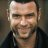@ APEX PREDATOR: does anyone have a cia of phonix wright trials and tribulation im trying to get it on my 3ds but...